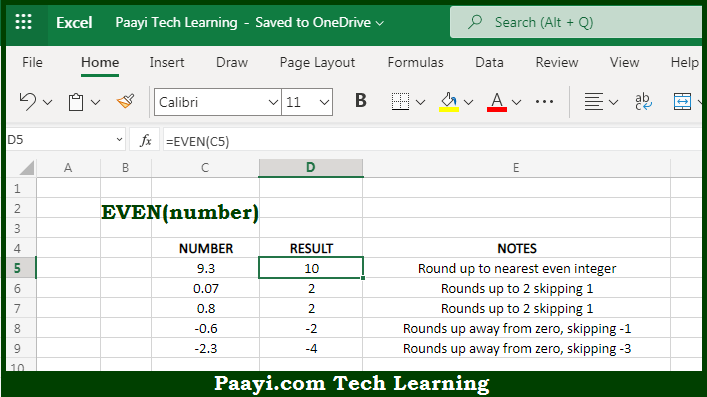# Learn How to Use Microsoft Excel EVEN Function

Written by | 0 Comments | 536 Views

In this article, you will learn how to use the Microsoft Excel EVEN function and its prime function in Microsoft Excel. You will also get to know the Microsoft Excel EVEN function return value and syntax with the help of some examples.

Microsoft Excel EVEN Function

The main function of the Microsoft Excel EVEN function is to round a number to the next even integer. So, with the help of the EVEN function, you can able to return the next EVEN integer after rounding up the given number. It should be noted that the EVEN function always rounds numbers up away from zero, which implies positive numbers become larger and negative numbers become smaller means more negative. So, with the help of the Microsoft Excel EVEN function you can easily round a number to the next even integer.

Return Value of EVEN Function

The return value will be the EVEN integer.

Syntax of EVEN Function

=EVEN(number)

Where the arguments:

• number: This is the number you want to round up to the even integer.

How to Use Microsoft Excel EVEN Function?So we know that the Microsoft Excel EVEN function is used to easily round up the number to the next even integer, leaving behind the odd integers in a way. It should be noted that the EVEN function always rounds numbers up away from zero, which implies positive numbers become larger and negative numbers become smaller means more negative. So, with the help of the Microsoft Excel EVEN function you can easily round a number to the next even integer.